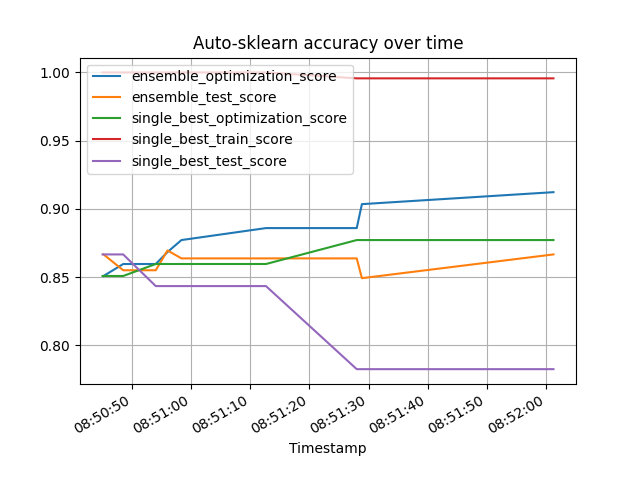# Performance-over-time plot¶

This example shows, how to use the performance_over_time_ attribute to plot the performance over train time. performance_over_time_ can contain multiple metrics within a pandas dataframe, namely:

• ensemble_optimization_score

• ensemble_test_score

• single_best_optimization_score

• single_best_test_score

• single_best_train_score

auto-sklearn can automatically encode categorical columns using a label/ordinal encoder. This example highlights how to properly set the dtype in a DataFrame for this to happen, and showcase how to input also testing data to autosklearn.

The X_train/y_train arguments to the fit function will be used to fit the scikit-learn model, whereas the X_test/y_test will be used to evaluate how good this scikit-learn model generalizes to unseen data (i.e. data not in X_train/y_train). Using test data is a good mechanism to measure if the trained model suffers from overfit, and more details can be found on evaluating estimator performance.

In order to provide *_test_score metrics, X_test and y_test must be provided to the AutoML-Model, as shown in this example.

There is also support to manually indicate the feature types (whether a column is categorical or numerical) via the argument feat_types from fit(). This is important when working with list or numpy arrays as there is no per-column dtype (further details in the example Feature Types).

```import time

import matplotlib.pyplot as plt
import numpy as np
import pandas as pd
import sklearn.model_selection
import sklearn.datasets
import sklearn.metrics

from smac.tae import StatusType

import autosklearn.classification
```

```# Using Australian dataset https://www.openml.org/d/40981.
# This example will use the command fetch_openml, which will
# as_frame=False, and manually creating the pandas DataFrame
X, y = sklearn.datasets.fetch_openml(data_id=40981, return_X_y=True, as_frame=False)

# bool and category will be automatically encoded.
# Targets for classification are also automatically encoded
# If using fetch_openml, data is already properly encoded, below
# is an example for user reference
X = pd.DataFrame(
data=X,
columns=['A' + str(i) for i in range(1, 15)]
)
desired_boolean_columns = ['A1']
desired_categorical_columns = ['A4', 'A5', 'A6', 'A8', 'A9', 'A11', 'A12']
desired_numerical_columns = ['A2', 'A3', 'A7', 'A10', 'A13', 'A14']
for column in X.columns:
if column in desired_boolean_columns:
X[column] = X[column].astype('bool')
elif column in desired_categorical_columns:
X[column] = X[column].astype('category')
else:
X[column] = pd.to_numeric(X[column])

y = pd.DataFrame(y, dtype='category')

X_train, X_test, y_train, y_test = sklearn.model_selection.train_test_split(
X, y, test_size=0.5, random_state=3
)
print(X.dtypes)
```

Out:

```A1         bool
A2      float64
A3      float64
A4     category
A5     category
A6     category
A7      float64
A8     category
A9     category
A10     float64
A11    category
A12    category
A13     float64
A14     float64
dtype: object
```

## Build and fit a classifier¶

```cls = autosklearn.classification.AutoSklearnClassifier(
per_run_time_limit=30,
)
cls.fit(X_train, y_train, X_test, y_test)
```

Out:

```/home/runner/work/auto-sklearn/auto-sklearn/autosklearn/metalearning/metalearning/meta_base.py:68: FutureWarning: The frame.append method is deprecated and will be removed from pandas in a future version. Use pandas.concat instead.
self.metafeatures = self.metafeatures.append(metafeatures)
/home/runner/work/auto-sklearn/auto-sklearn/autosklearn/metalearning/metalearning/meta_base.py:72: FutureWarning: The frame.append method is deprecated and will be removed from pandas in a future version. Use pandas.concat instead.
self.algorithm_runs[metric].append(runs)

```

## Get the Score of the final ensemble¶

```predictions = cls.predict(X_test)
print("Accuracy score", sklearn.metrics.accuracy_score(y_test, predictions))
```

Out:

```Accuracy score 0.8753623188405797
```

## Plot the ensemble performance¶

The performance_over_time_ attribute returns a pandas dataframe, which can be directly used for plotting

```poT = cls.performance_over_time_
poT.plot(
x='Timestamp',
kind='line',
legend=True,
title='Auto-sklearn accuracy over time',
grid=True,
)
plt.show()
```Total running time of the script: ( 2 minutes 2.483 seconds)

Gallery generated by Sphinx-Gallery